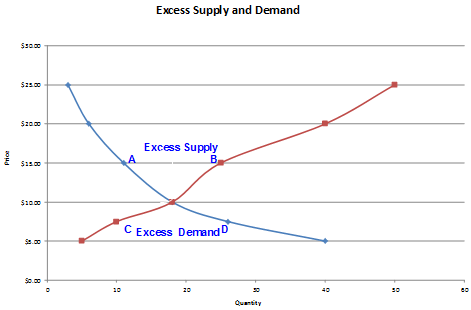# Excess Demand and Excess Supply

Based on the demand and supply curve, the market forces drive the price to its equilibrium level.

There are two possibilities: 1) Excess Demand or 2) Excess Supply

Excess supply is the situation where the price is above its equilibrium price. The quantity willing supplied by the producers is higher than the quantity demanded by the consumers.

Excess demand is the situation where the price is below its equilibrium price. The quantity supplied is lower than the quantity demanded by the consumers.

The following chart illustrates the excess demand and excess supply.In each of these situations market forces will interact to drive the prices to its equilibrium level.

When we have higher prices and excess supply, manufacturers will have excess inventories and the competition among manufacturers will put the downward pressure on price as there will be some suppliers who will be willing to supply at lower prices. As prices fall, the consumer demand will increase until it finally settles at the equilibrium price.

When we have lower prices and excess demand, there will shortage of goods, putting an upward pressure on the price as there will be more buyers chasing the available goods. As price increases the suppliers will start producing more but the demand from buyers will decrease. This will drive the price and quantity to its equilibrium level.

Calculating Excess Supply and Demand

Let’s say we have the following demand and supply functions:

Qd = 415,000 – 1,200P

Qs = 40,000+150P

The equilibrium price can be calculated by equating the two functions and solving for P

415,000 – 1,200P = 40,000+150P

P = 375,000/1350 = 277.78

We will have excess supply when price is above 277.78 and excess demand when price is below 277.78.

At this price the quantity demanded and supplied is 81,667.

At P=300, the quantity supplied will be = 40,000+150*300 = 85,000. The excess supply is 85,000 – 81,667 = 3,333

At P = 200, the quantity demanded is = 415,000 – 1,200*200 = 175,000. The excess demand is 175,000 – 81,667 = 93,333.